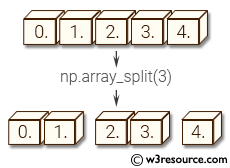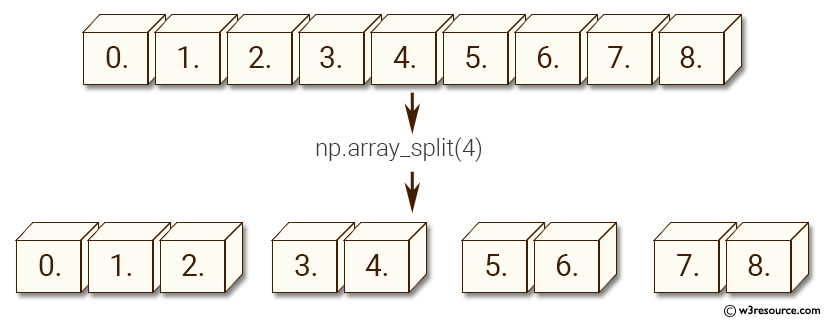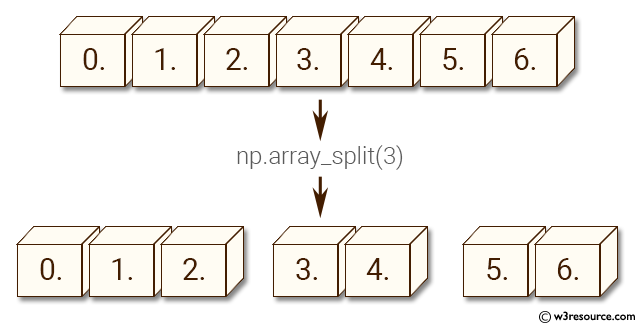# NumPy Array manipulation: array_split() function

## numpy.array_split() function

The array_split() function split an given array into multiple sub-arrays.
The only difference between these functions is that array_split allows indices_or_sections to be an integer that does not equally divide the axis. For an array of length l that should be split into n sections, it returns l % n sub-arrays of size l//n + 1 and the rest of size l//n.

Syntax:

`numpy.array_split(ary, indices_or_sections, axis=0)`Version: 1.15.0

Parameter:

Name Description Required /
Optional
ary Input array. Required
indices_or_sections Indices or sections. Required
axis The axis along which values are appended. If axis is not given, both arr and values are flattened before use. Optional

Return value:

Example-1: numpy.array_split()

``````>>> import numpy as np
>>> a = np.arange(9.0)
>>> np.array_split(a, 4)
[array([ 0.,  1.,  2.]), array([ 3.,  4.]), array([ 5.,  6.]), array([ 7.,  8.])]
``````

Pictorial Presentation:Example-2: numpy.array_split()

``````>>> import numpy as np
>>> a = np.arange(7.0)
>>> np.array_split(a, 3)
[array([ 0.,  1.,  2.]), array([ 3.,  4.]), array([ 5.,  6.])]
``````

Pictorial Presentation:Python - NumPy Code Editor:

Previous: split()
Next: dsplit()

﻿